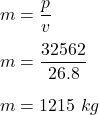## A car that drives at 26.8 m/s has a momentum of 32,562 kg-m/s. What is the mass of the car?

Question

A car that drives at 26.8 m/s has a momentum of 32,562 kg-m/s. What is the mass of the car?

in progress 0
5 months 2021-08-30T06:58:42+00:00 1 Answers 4 views 0

m = 1215 kg

Explanation:

It is given that,

Speed of a car is 26.8 m/s

Momentum of the car is 32,562 kg-m/s

We need to find the mass of the car. Momentum of the car is given by :

p = mvSo, the mass of the car is 1215 kg.